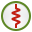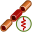# LD Card

This card specifies a distributed resistive, capacitive or inductive loading or a series combination of these loads for a segment(s).

On the Source/Load tab, in the Loads / networks group, click theLoad icon. From the drop-down list, click theDistributed load (LD) icon.

## Parameters:

Define/replace a load at a segment
Define a load with the following parameters.
Remove all LD type loads previously defined
This LD card does not define a load, but rather all previously defined LD loads are deleted. All the other input parameters of this card are ignored.
Resistance:
The distributed resistance in $\frac{\Omega }{m}$ .
Inductance
The distributed inductance in $\frac{H}{m}$ .
Capacitance
The distributed capacitance in $\frac{F}{m}$ .

The combined impedance of the segment with length $l$ is then

(1) ${Z}_{s}=\left(R\text{´}+j\omega L\text{´}+\frac{1}{j\omega C\text{´}}\right)\cdot l$

It should be noted that if the Capacitance (F/m) is left empty, it is treated as infinite, so that it does not contribute to the impedance.

The LD card may be combined with the LP, LS, LZ and the SK cards, but only one LD card may be used per label. If a second LD card is used, it replaces the values entered by the first card. This card has no significance for surface elements, even when these are assigned the same label.